Introduction

There are numerous ways to solve quadratic equations. This unit will cover graphing, using square roots, factoring, the quadratic formula, and completing the square.

When solving a quadratic equation, there will be two, one or no real solutions. When there are no real solutions the solutions can be represented by the imaginary number i. Since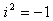, the complex number i is defined as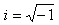. This number can help to define any square root of a negative number.

Example 1

Simplify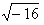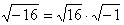, the square roots can be simplified to 4i.

Example 2

Simplify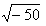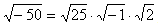, the square roots can be simplified to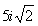.

Listen